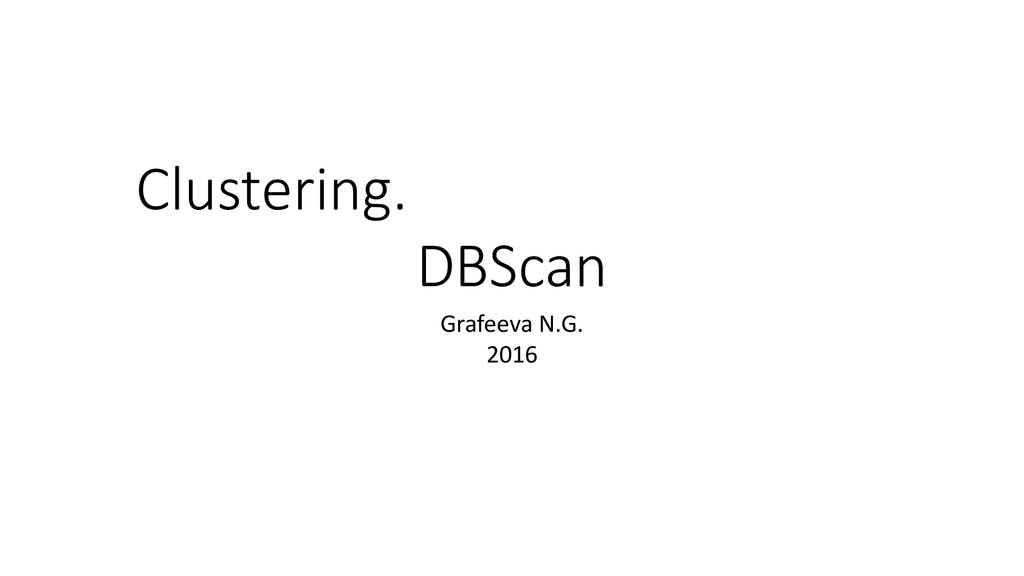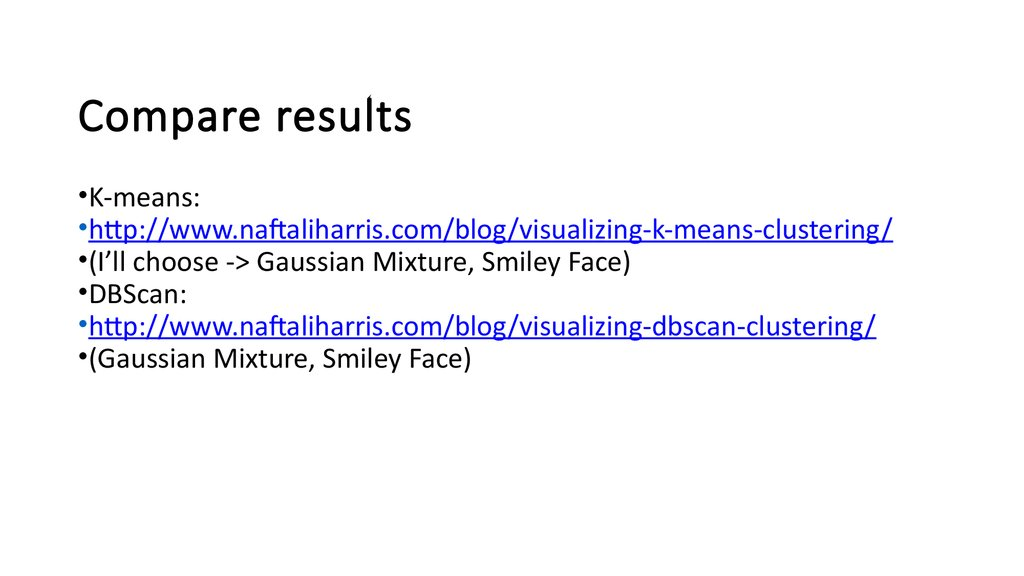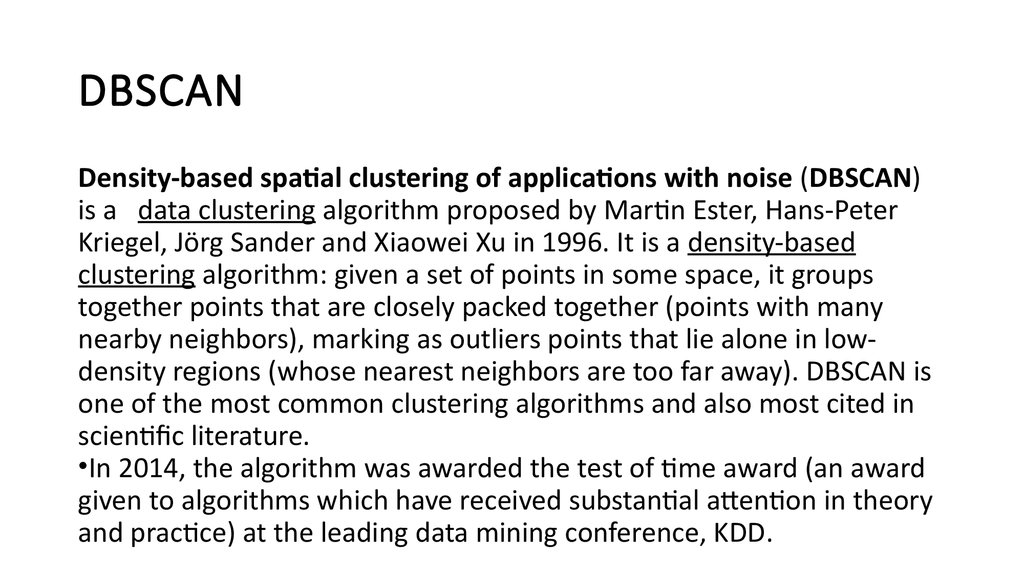# Clustering. DBScan

Clustering.
DBScan
Grafeeva N.G.
2016

## 2.

Compare results
•K-means:
•http://www.naftaliharris.com/blog/visualizing-k-means-clustering/
•(I’ll choose -> Gaussian Mixture, Smiley Face)
•DBScan:
•http://www.naftaliharris.com/blog/visualizing-dbscan-clustering/
•(Gaussian Mixture, Smiley Face)

## 3.

DBSCAN
Density-based spatial clustering of applications with noise (DBSCAN)
is a data clustering algorithm proposed by Martin Ester, Hans-Peter
Kriegel, Jörg Sander and Xiaowei Xu in 1996. It is a density-based
clustering algorithm: given a set of points in some space, it groups
together points that are closely packed together (points with many
nearby neighbors), marking as outliers points that lie alone in lowdensity regions (whose nearest neighbors are too far away). DBSCAN is
one of the most common clustering algorithms and also most cited in
scientific literature.
•In 2014, the algorithm was awarded the test of time award (an award
given to algorithms which have received substantial attention in theory
and practice) at the leading data mining conference, KDD.

## 4.

Preliminary
Consider a set of points in some space to be clustered. For the purpose
of DBSCAN clustering, the points are classified as core points, (density-)
reachable points and outliers (noise), as follows:
•A point p is a core point if at least minPts points are within distance ε
of it, and those points are said to be directly reachable from p.
•A point q is reachable from p if there is a path p1, ..., pn with p1 = p and
pn = q, where each pi+1 is directly reachable from pi (so all the points on
the path must be core points, with the possible exception of q).
•All points not reachable from any other point are outliers.

## 5. Preliminary

• If p is a core point, then it forms a cluster together with all
points
Preliminary
• (core or non-core) that are reachable from it. Each cluster
contains at
• least one core point; non-core points can be part of a cluster,
but they
• form its "edge", since they cannot be used to reach more
points.

## 6. Preliminary

Two points p and q are density-connected if there is a point o such that
both p and q are density-reachable from o. Density-connectedness is
symmetric.
A cluster satisfies two properties:
•All points within the cluster are mutually density-connected.
•If a point is density-reachable from any point of the cluster, it is part of
the cluster as well.

## 7. Example

In this diagram, minPts = 3. Point A and
the other red points are core points,
because at least three points surround it in
an ε radius. Because they are all
reachable from one another, they form a
single cluster. Points B and C are not core
points, but are reachable from A (via other
core points) and thus belong to the cluster
as well. Point N is a noise point that is
neither a core point nor density-reachable.

## 8.

Algorithm
DBSCAN requires two parameters: ε (eps) and the minimum number of
points required to form a dense region (minPts). It starts with an
arbitrary starting point that has not been visited. This point's εneighborhood is retrieved, and if it contains sufficiently many points, a
cluster is started. Otherwise, the point is labeled as noise. Note that
this point might later be found in a sufficiently sized ε-environment of a
different point and hence be made part of a cluster.

## 9. Algorithm

If a point is found to be a dense part of a cluster, its ε-neighborhood is
also part of that cluster. Hence, all points that are found within the εneighborhood are added, as is their own ε-neighborhood when they
are also dense. This process continues until the density-connected
cluster is completely found. Then, a new unvisited point is retrieved
and processed, leading to the discovery of a further cluster or noise.
The algorithm can be expressed as follows, in pseudocode following
the original published nomenclature.

Main procedure

## 11.

Procedure expandCluster

## 12.

Procedure regionQuery

## 13.

Note
The algorithm can be simplified by merging the per-point "has been
visited" and "belongs to cluster C" logic, as well as by inlining the
contents of the "expandCluster" subroutine, which is only called from
one place. These simplifications have been omitted from the above
pseudocode in order to reflect the originally published version.
Additionally, the regionQuery function need not return P in the list of
points to be visited, as long as it is otherwise still counted in the local
density estimate.

## 14.

Complexity
DBSCAN visits each point of the database, possibly multiple times (e.g.,
as candidates to different clusters). For practical considerations,
however, the time complexity is mostly governed by the number of
regionQuery invocations. DBSCAN executes exactly one such query for
each point, and if an indexing structure is used that executes a
neighborhood query in O(log n), an overall average runtime complexity
of O(n log n) is obtained (if parameter ε is chosen in a meaningful way,
i.e. such that on average only O(log n) points are returned). Without
the use of an accelerating index structure, or on degenerated data (e.g.
all points within a distance less than ε), the worst case run time
complexity remains O(n²).

## 15.

Parameter estimation (minPts)
Ideally, minPts is the desired minimum cluster size. Otherwise a
minimum minPts can be derived from the number of dimensions D in
the data set, as minPts≥D+ 1. The low value of minPts = 1 does not
make sense, as then every point on its own will already be a cluster.
With minPts ≤ 2, the result will be the same as of hierarchical
clustering. Therefore, minPts must be chosen at least 3. However, larger
values are usually better for data sets with noise. The larger the data
set, the larger the value of minPts should be chosen.

## 16.

Parameter estimation (ε)
If ε is chosen much too small, a large part of the data will not be
clustered; whereas for a too high value of ε, clusters will merge and the
majority of objects will be in the same cluster. In general, small values
of ε are preferable, and as a rule of thumb only a small fraction of
points should be within this distance of each other.
•Distance function: The choice of distance function is tightly coupled to
the choice of ε, and has a major impact on the results. In general, it will
be necessary to first identify a reasonable measure of similarity for the
data set, before the parameter ε can be chosen.

## 17.

•DBSCAN does not require to specify the number of clusters in the data a priori, as
opposed to k-means.
•DBSCAN can find arbitrarily shaped clusters. It can even find a cluster completely
surrounded by (but not connected to) a different cluster. Due to the MinPts
parameter, the so-called single-link effect (different clusters being connected by a
thin line of points) is reduced.
•DBSCAN can find outliers.
•DBSCAN requires just two parameters and is mostly insensitive to the ordering of
the points in the database. (However, points sitting on the edge of two different
clusters might swap cluster membership if the ordering of the points is changed)
•The parameters minPts and ε can be set by an expert, if the data is well
understood.

## 18.

•DBSCAN is not entirely deterministic: border points that are reachable from more
than one cluster can be part of either cluster, depending on the order the data is
processed.
•The quality of DBSCAN depends on the distance measure used in the function
regionQuery(P,ε). The most common distance metric used is Euclidean distance.
Especially for high-dimensional data, this metric can be rendered almost useless
due to the so-called "Curse of dimensionality", making it difficult to find an
appropriate value for ε. This effect, however, is also present in any other algorithm
based on Euclidean distance.
•DBSCAN cannot cluster data sets well with large differences in densities, since the
minPts-ε combination cannot then be chosen appropriately for all clusters.
•If the data and scale are not well understood, choosing a meaningful distance
threshold ε can be difficult.

• Nothing…